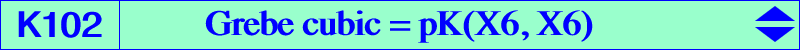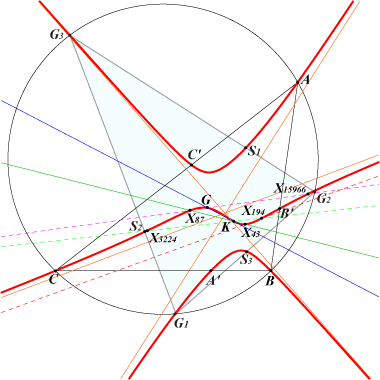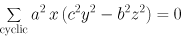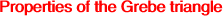X(1), X(2), X(6), X(43), X(87), X(194), X(3224) excenters A', B', C' : vertices of the cevian triangle of K foci of the K-ellipse (inellipse with center K when the triangle ABC is acutangle) G1, G2, G3 : vertices of the Grebe triangle (see below) S1, S2, S3 : projections of G on the sidelines of the Grebe triangle, these points on the bicevian conic C(G, K). imaginary foci of the Brocard ellipse i.e. common points of the Brocard axis and the Kiepert hyperbola other points in the table belowThe Grebe cubic is pK(X6, X6) i.e. the isogonal pK with pivot K = X(6), the Lemoine point. See also Z+(L)60. It is anharmonically equivalent to the McCay cubic K003. The symbolic substitution SS{a -> √a} transforms K102 into K101. Locus properties : locus of point P such that the trilinear polars of P and its isogonal conjugate P* are parallel. Thus K102 is a member of the class CL007 of cubics. Now, if we replace trilinear polars by orthotransversals, we obtain the sextic Q021. locus of M such that the circumanticevian triangle of M and the anticevian triangle of its isogonal conjugate M* are perspective (together with another isogonal nK with root G of the class CL020). locus of point P such that the polars of P and P* in the bicevian conic C(P, P*) are parallel. locus of point P such that the center of the bicevian conic C(P, P*) lies on the line PP* (Francisco Javier García Capitán). The locus of this center is K437, a nodal cubic with node K. Kjp = K024 is an isogonal nK0 which meets its asymptotes on a parallel to the trilinear polar of its root. This is true for any nK0(X6, P) with P on the Grebe cubic K102. See also K082. see the related CL064. K102 meets the Steiner ellipse again at three points and the tangents at these points are concurrent at X = X(14897). The three points G1, G2, G3 (other than A, B, C) where K102 meets the circumcircle of ABC are the vertices of a triangle we call the Grebe triangle. These points are the centers of the three isogonal central circular nK0. See isogonal nK0.Group law on the Grebe cubic As in the page K002, a group law is defined on K102 with neutral element X(6). For P, Q on K102, P + Q is the isogonal conjugate of the third point of K102 on the line PQ. Each center P on K102 is associated with an integer n. Three points on K102 are collinear if and only if the sum of the corresponding integers is 2. Q is the tangential of P.n -6 -5 -4 -3 -2 -1 0 1 2 3 4 5 6 P X(15963) X(15964) X(15965) X(15966) X(194) X(43) X(6) X(1) X(2) X(87) X(3224) X(15967) X(15968) Q X(15968) X(3224) X(2) X(6) X(194) X(15965) X(15963)If P and P' are two points with associated integers n and n' then : • P and P' are X(6)-Ceva conjugates if and only if n + n' = 0, in which case X(2), P, P' are collinear, • P and P' are isogonal conjugates if and only if n + n' = 2, in which case X(6), P, P' are collinear, • P and P' are X(2)-cross conjugates if and only if n + n' = 4, in which case X(194), P, P' are collinear. The tangential Q of P corresponds to the integer 2 - 2n hence the isogonal conjugate of Q corresponds to 2n.The Grebe triangle G1G2G3 is acutangle in every reference triangle ABC. 1. These three points G1, G2, G3 lie on the circumcircle and on several remarkable rectangular hyperbolas passing through : X(i) for i in {2, 3, 6, 83, 99, 194, 3413, 3414, 5652, 6033, 12177, 14535, 15652, 16508, 18773, 18774, 22498, 22561, 22594, 22623, 22664, 22683, 22685, 22687, 22689, 22716, 22718, 31958, 33346, 33347, 33380, 33381, 33408, 33409} which is the Jerabek hyperbola of the Grebe triangle and also the Kiepert hyperbola of the reflected first Brocard triangle. See Table 32. X(6), X(110), X(182), X(206), X(1176), X(1386), X(2574), X(2575) X(6), X(30), X(230), X(476), X(523), X(1995) X(6), X(32), X(112), X(251), X(3162) X(6), X(511), X(512), X(805), X(3231) X(6), X(513), X(517), X(901), X(2243), X(3246) Note that all these hyperbolas contain X(6) since it is the orthocenter of G1G2G3 and that the Euler line of G1G2G3 is actually the Brocard axis of ABC. 2. The inconic with center X(3589) and perspector X(83) is also inscribed in G1G2G3. See the related cubic K644. Note that this inconic and the bicevian conic C(G, K) mentioned above are concentric. 3. G1, G2, G3 lie on several listed cubics such as K177, K281, K642, K643, K644, K729, K731 and also the isogonal transform of K527 which is spK(X1352, X5). More generally, the isogonal transform of any spK(K, Q) of CL055 contains G1, G2, G3 and X(6). This latter cubic is a pK if and only if the complement of Q lies on the central cubic K140 in which case its pole, pivot, isopivot lie on K1258 = pK(X251 x X32, X251), K644, K177 respectively. Examples of such pKs : K1258 = pK(X251 x X3, X251), pK(X1176 x X25, X1176), pK(X25, X8743), pK(X206 x X4, X4). See also Table 57. 4. The Jerabek and Stammler hyperbolas of the Grebe triangle are homothetic to the Kiepert hyperbola of ABC. The second conic passes through X(i) for i in {3, 381, 1676, 1677, 3413, 3414, 12188, 14535}. *** The following table shows several centers of the Grebe Triangle and their counterparts in ABC (Chris van Tienhoven, Peter Moses). Note that X(3) in yellow remains unchanged.X(2) in GrebeTriangle = X(5085) in ABC X(1141) in GrebeTriangle = X(110) in ABC X(3) in GrebeTriangle = X(3) in ABC X(1154) in GrebeTriangle = X(30) in ABC X(4) in GrebeTriangle = X(6) in ABC X(1157) in GrebeTriangle = X(23) in ABC X(5) in GrebeTriangle = X(182) in ABC X(1173) in GrebeTriangle = X(83) in ABC X(20) in GrebeTriangle = X(1350) in ABC X(1291) in GrebeTriangle = X(842) in ABC X(24) in GrebeTriangle = X(1384) in ABC X(1304) in GrebeTriangle = X(5970) in ABC X(30) in GrebeTriangle = X(511) in ABC X(1487) in GrebeTriangle = X(1176) in ABC X(54) in GrebeTriangle = X(2) in ABC X(1493) in GrebeTriangle = X(5) in ABC X(74) in GrebeTriangle = X(99) in ABC X(1510) in GrebeTriangle = X(523) in ABC X(107) in GrebeTriangle = X(729) in ABC X(1614) in GrebeTriangle = X(183) in ABC X(110) in GrebeTriangle = X(98) in ABC X(2070) in GrebeTriangle = X(2080) in ABC X(125) in GrebeTriangle = X(5026) in ABC X(2383) in GrebeTriangle = X(112) in ABC X(140) in GrebeTriangle = X(5092) in ABC X(2574) in GrebeTriangle = X(3414) in ABC X(185) in GrebeTriangle = X(3734) in ABC X(2575) in GrebeTriangle = X(3413) in ABC X(186) in GrebeTriangle = X(187) in ABC X(2777) in GrebeTriangle = X(5969) in ABC X(195) in GrebeTriangle = X(381) in ABC X(2914) in GrebeTriangle = X(115) in ABC X(378) in GrebeTriangle = X(5024) in ABC X(3484) in GrebeTriangle = X(5971) in ABC X(381) in GrebeTriangle = X(5050) in ABC X(3518) in GrebeTriangle = X(32) in ABC X(382) in GrebeTriangle = X(1351) in ABC X(3520) in GrebeTriangle = X(574) in ABC X(403) in GrebeTriangle = X(2030) in ABC X(3543) in GrebeTriangle = X(5102) in ABC X(476) in GrebeTriangle = X(2698) in ABC X(3574) in GrebeTriangle = X(597) in ABC X(477) in GrebeTriangle = X(805) in ABC X(3627) in GrebeTriangle = X(576) in ABC X(523) in GrebeTriangle = X(512) in ABC X(3830) in GrebeTriangle = X(5093) in ABC X(539) in GrebeTriangle = X(1503) in ABC X(3853) in GrebeTriangle = X(5097) in ABC X(546) in GrebeTriangle = X(575) in ABC X(5663) in GrebeTriangle = X(2782) in ABC X(550) in GrebeTriangle = X(3098) in ABC X(6000) in GrebeTriangle = X(538) in ABC X(526) in GrebeTriangle = X(804) in ABC X(930) in GrebeTriangle = X(74) in ABC X(933) in GrebeTriangle = X(111) in ABC X(1113) in GrebeTriangle = X(1379) in ABC X(1114) in GrebeTriangle = X(1380) in ABC